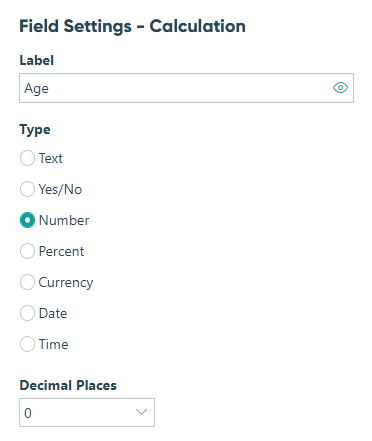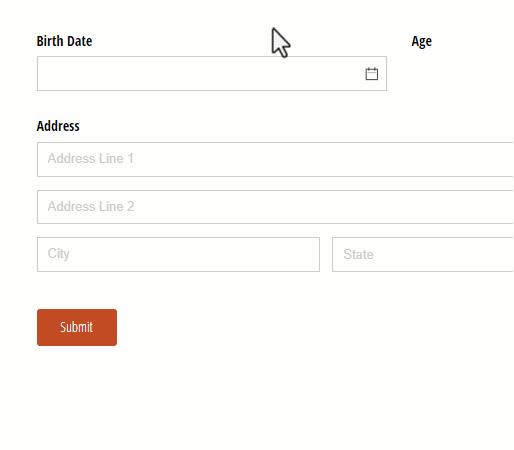# How do I automatically calculate someone's age?

To calculate someone’s age based on their birth date:

2. Add a Calculation field labelled Age, set to the Number type with 0 decimal places.3. Insert one of the following expressions as your calculation:

• Option 1: Calculate age in years using `= DateTime.Today.Year - BirthDate.Year + (if DateTime.Today.Month < BirthDate.Month or (DateTime.Today.Month = BirthDate.Month and DateTime.Today.Day < BirthDate.Day) then -1 else 0)`
• Option 2: Calculate age in months using `=DateTime.Today.Year * 12 + DateTime.Today.Month - BirthDate.Year * 12 - BirthDate.Month + (DateTime.Today.Day < BirthDate.Day ? -1 : 0)`

Now, when a user selects their date of birth, the calculation field will automatically determine their age.